# Upcoming Important Dates

Tuesday, February 23 - Special LSC Meeting
Tuesday, February 23 - FON Board Meeting
Martes, 24 Febrero - Hora de Conversación en Español
Tuesday, March 9 - Financial Aid Presentation: Juniors and Sophomores

 table div table+table+table+table div table{width:100%;padding:0}table div table+table+table+table div table img{width:96.23%;padding:0;float:none}table div table+table+table+table div table td{width:100%;padding:0 1.88% 18px}/* styles */# Additional Info Below

▪ Special LSC Meeting Tuesday, February 23, 6:00 pm
▪ A Message from the LSC Chair About Principal Mest
▪ Friends of Northside Board Meeting, February 23, 7:00 pm
▪ Hora de Conversación en Español - Martes de Febrero 24, 7:00 pm
▪ FON Spring Benefit Call for Student Artwork, March 1 Deadline
▪ Financial Aid Presentation Planned for Juniors and Sophomores on Tuesday, March 9
▪ College Planning Night: Follow-up
▪ Class of 2022 - Important Dates
▪ Update: Postsecondary Representative Visits
▪ Counseling Department: ILP Naviance Curriculum
▪ College Scholarship Notifications
▪ SAT Updates from College Board
▪ Available Now: Custom NCP Brick With Your Personalized Message for Your Student
▪ Any Questions About SchoolCNXT?
▪ Winter Sports Update
▪ Photo Submissions Requested for NCP Stampede!
▪ Yearbook Ads for Seniors Available
▪ Update on School Portraits for 9th, 10th, and 11th Graders
▪ Order Your 2020-2021 NCP Yearbook
▪ Ways for Parents to Stay in Touch
 ▪ Special LSC Meeting Tuesday, February 23, 6:00 pm
 ▪ A Message from the LSC Chair About Principal Mest
 ▪ Friends of Northside Board Meeting, February 23, 7:00 pm
 ▪ Hora de Conversación en Español - Martes de Febrero 24, 7:00 pm
 ▪ FON Spring Benefit Call for Student Artwork, March 1 Deadline
 ▪ Financial Aid Presentation Planned for Juniors and Sophomores on Tuesday, March 9
 ▪ College Planning Night: Follow-up
 ▪ Class of 2022 - Important Dates
 ▪ Update: Postsecondary Representative Visits
 ▪ Counseling Department: ILP Naviance Curriculum
 ▪ College Scholarship Notifications
 ▪ SAT Updates from College Board
 ▪ Available Now: Custom NCP Brick With Your Personalized Message for Your Student
 ▪ Any Questions About SchoolCNXT?
 ▪ Winter Sports Update
 ▪ Photo Submissions Requested for NCP Stampede!
 ▪ Yearbook Ads for Seniors Available
 ▪ Update on School Portraits for 9th, 10th, and 11th Graders
 ▪ Order Your 2020-2021 NCP Yearbook
 ▪ Ways for Parents to Stay in Touch
 table div table+table+table+table+table+table div table{width:100%;padding:0}table div table+table+table+table+table+table div table img{width:96.23%;padding:0;float:none}table div table+table+table+table+table+table div table td{width:100%;padding:0 1.88% 18px}/* styles */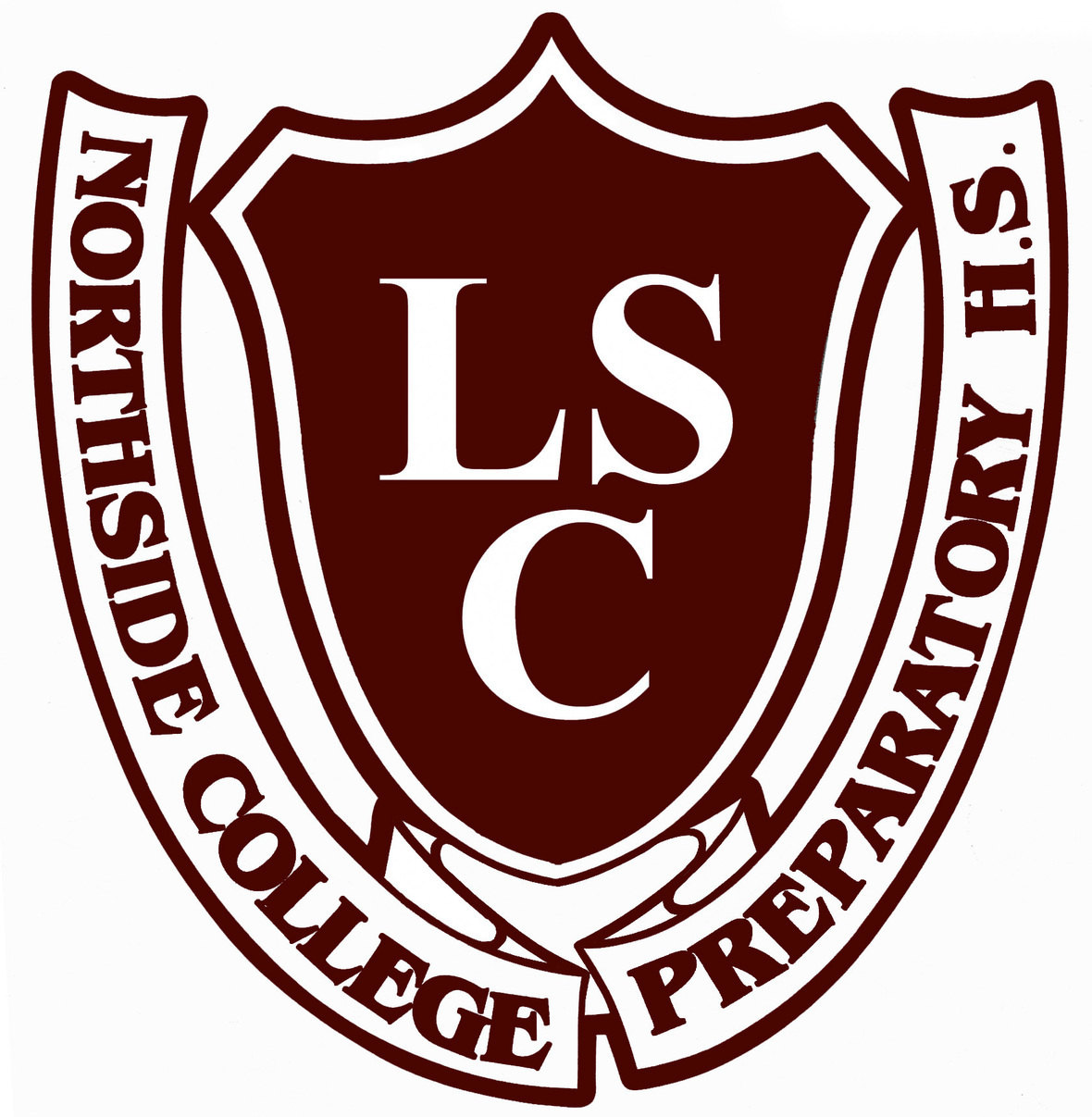# Special LSC Meeting

Tuesday, February 23
6:00 pm Zoom Meeting

To view the agenda for this month's meeting, click HERE.

## LSC Meeting Zoom Link

To join the meeting on Zoom, please click here or dial +13126266799.

Both the Zoom link and the agenda can be found on the Northside website here.

Meeting ID: 896 8543 3325
Passcode: 088112

Meeting ID: 896 8543 3325
Passcode: 088112

## A Message from the LSC Chair

By now you have heard the news that Kelly Mest’s departing Northside to become the Deputy Chief of Network 14. Please join me in congratulating her as she takes this next step in her professional career. My children had the good fortune of her being their Principal these past seven-plus years and I have been privileged to work with her as a member of the LSC for the past four years. She is a dedicated, thoughtful leader who always put the students first. Focused not only on academic excellence but developing the whole student both socially and emotionally, developing future citizens that care about making a positive impact on their community.

The LSC is ready to build on Kelly’s foundation of excellence by conducting a successful search for the next Principal who will be deserving of leading such a wonderful community of families, students, and faculty. As we begin the process, we will be reaching out to you in the coming weeks with updates. Please feel free to contact us at northsidecollegepreplsc@gmail.com.

Shari Massey
Northside College Prep LSC Chair

 table div table+table+table+table+table+table+table+table div table{width:100%;padding:0}table div table+table+table+table+table+table+table+table div table img{width:96.23%;padding:0;float:none}table div table+table+table+table+table+table+table+table div table td{width:100%;padding:0 1.88% 18px}/* styles */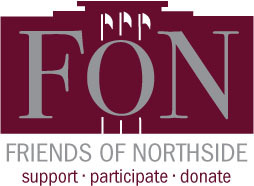# Friends of Northside

Tuesday, February 23
7:00 pm Google Meet

Please join Friends of Northside for their upcoming meeting.

Friends of Northside (FON) is the “one-stop-shop” for NCP families to get involved. FON facilitates communication between parents and the school, organizes parent volunteers, plans and sponsors social/educational events, and raises much-needed funds to bridge the gap due to CPS budget cuts. FON supports the school’s mission to maintain and enhance the learning experience for all students of Northside College Prep High School.

To find out more about Friends of Northside, please visit their website HERE.

 table div table+table+table+table+table+table+table+table+table+table div table{width:100%;padding:0}table div table+table+table+table+table+table+table+table+table+table div table img{width:96.23%;padding:0;float:none}table div table+table+table+table+table+table+table+table+table+table div table td{width:100%;padding:0 1.88% 18px}/* styles */# Hora de Conversación en Español

Para los padres interesados ​​en conocer a otros padres, hacer preguntas y discutir temas de interés relacionados con Northside College Prep, los invitamos a participar en una discusión informal el miércoles 24 de febrero a las 7:00 pm. Pueden conectarse por Zoom o por teléfono.

Sandra Morales-Mirque, nueva miembra del Consejo Escolar Local, estará facilitando la conversación. Otros miembros de Amigos de Northside estarán disponibles para contestar preguntas.

Se enviará un enlace de Zoom Meeting en el boletín de la próxima semana.

Si tiene alguna pregunta puede enviarla con anticipación a: sandra.mirque@gmail.com.

 table div table+table+table+table+table+table+table+table+table+table+table+table+table div table{width:100%;padding:0}table div table+table+table+table+table+table+table+table+table+table+table+table+table div table img{width:96.23%;padding:0;float:none}table div table+table+table+table+table+table+table+table+table+table+table+table+table div table td{width:100%;padding:0 1.88% 18px}/* styles */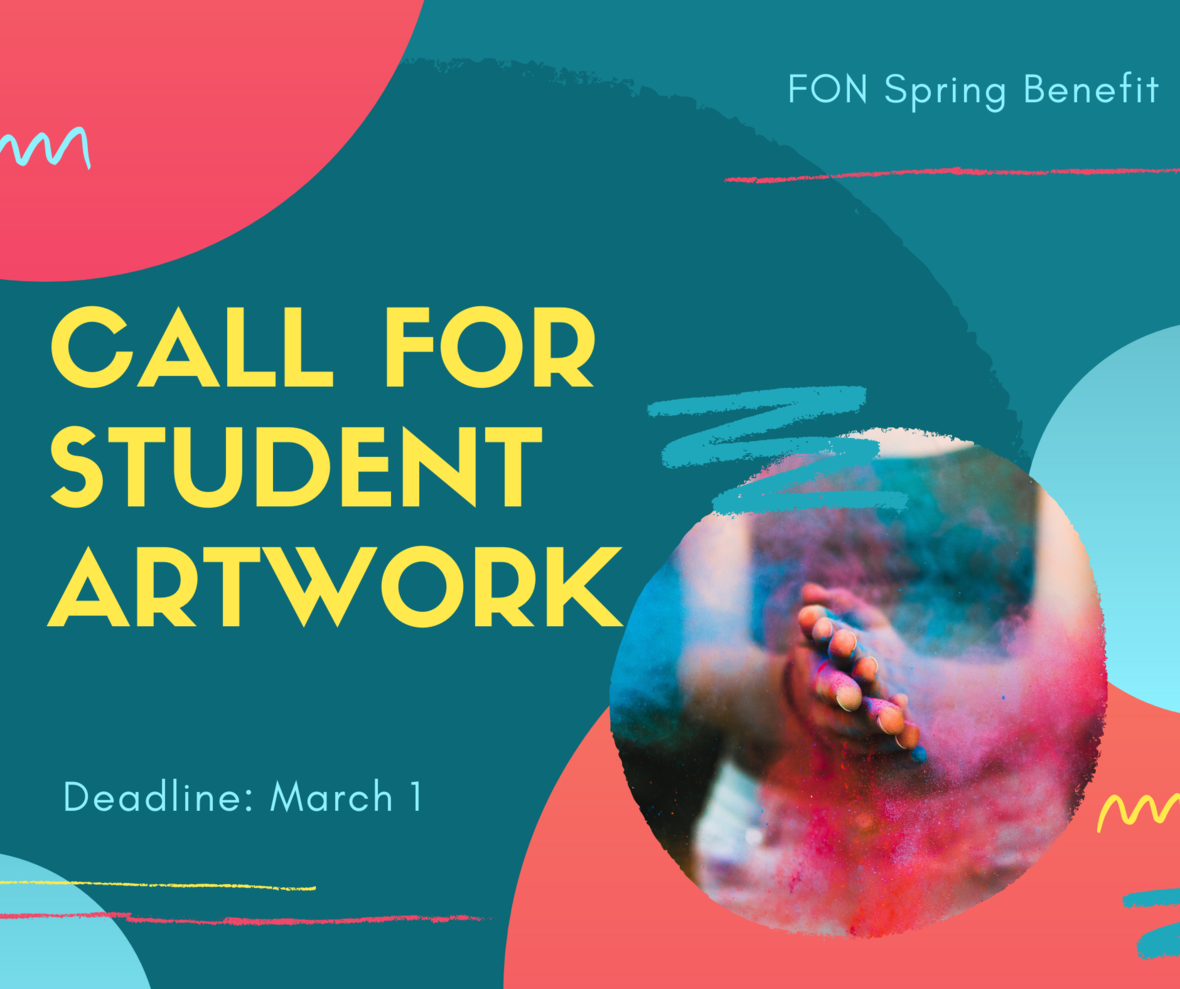# FON Spring Benefit Call for Student Artwork: Submissions Due by March 1

Friends of Northside is looking for NCP students to help them visually illustrate their Spring Virtual Fundraising Event theme: "Together but Apart." The past year has been one like no other, and they would like to showcase the resilience, strength, and resourcefulness that our students, faculty, and parents have demonstrated and which continue to make Northside a place that exemplifies what is possible in public education.

Students have been asked via NCP email to submit their creative interpretation of the event theme, “Together but Apart” as a two-dimensional drawing, painting, computer illustration, collage, or another medium that can be scanned flat and sent as a high-resolution color 300 dpi jpg, pdf or eps file. Selected artwork will be featured in marketing materials and communications before and during the event.

The email contains a Google Form to submit their artwork. Submissions are due by Monday, March. 1.

 table div table+table+table+table+table+table+table+table+table+table+table+table+table+table+table+table div table{width:100%;padding:0}table div table+table+table+table+table+table+table+table+table+table+table+table+table+table+table+table div table img{width:96.23%;padding:0;float:none}table div table+table+table+table+table+table+table+table+table+table+table+table+table+table+table+table div table td{width:100%;padding:0 1.88% 18px}/* styles */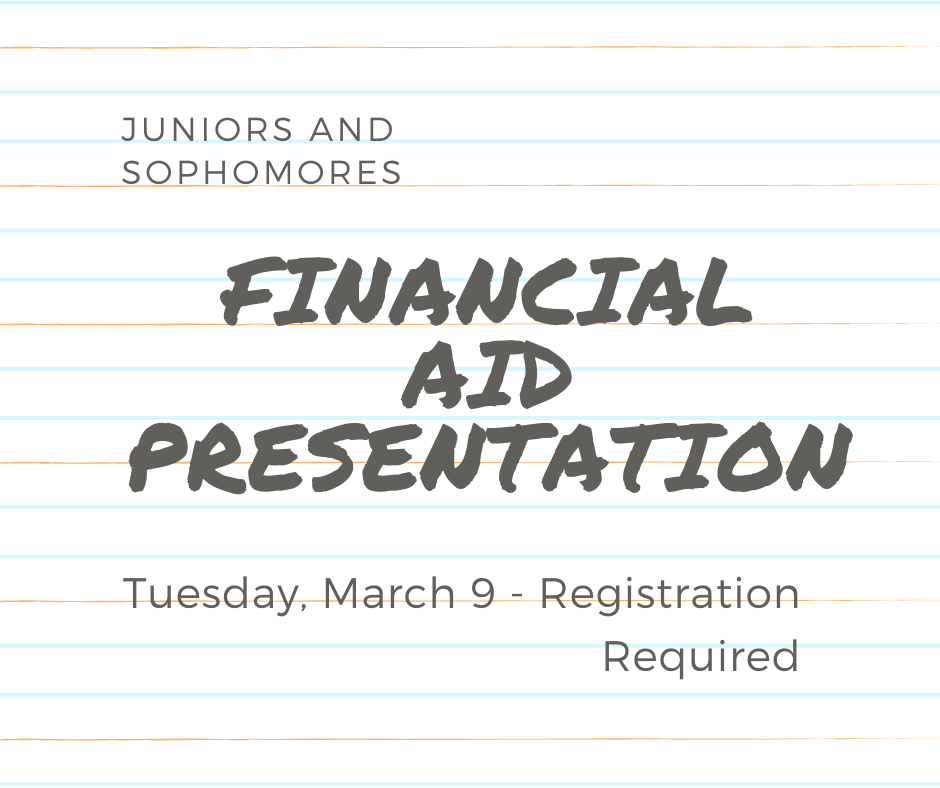# Financial Aid Presentation Planned for Juniors and Sophomores on Tuesday, March 9

Hello, Junior and Sophomore Parents,

On Tuesday, March 9, from 6:00 pm - 8:00 pm, the Northside College Prep Counseling Department will host a virtual financial aid presentation for students and parents and/or guardians.

This presentation is being offered to help your family prepare to navigate the financial aid process. During the presentation, the presenters will outline the financial aid process for the Free Application for Federal Student Aid (FAFSA), R.I.S.E. Application for undocumented and transgendered students, and need-based financial aid.

To ensure we are meeting the needs of the Northside Prep community, we are offering presentations in English and Spanish. (Click here) to register for the virtual financial aid presentation. Once we receive your registration, you will receive a Google Meeting invitation by Friday, March 6.

If you have any questions or concerns, feel free to contact Ms. Moore at mcmoore6@cps.edu.

 table div table+table+table+table+table+table+table+table+table+table+table+table+table+table+table+table+table+table+table div table{width:100%;padding:0}table div table+table+table+table+table+table+table+table+table+table+table+table+table+table+table+table+table+table+table div table img{width:96.23%;padding:0;float:none}table div table+table+table+table+table+table+table+table+table+table+table+table+table+table+table+table+table+table+table div table td{width:100%;padding:0 1.88% 18px}/* styles */# College Planning Night: Follow-up

Junior Students and Parents,

Thank you for attending the Northside Prep, Junior College Planning Night on Monday, February 8. The counseling team is looking forward to discussing your postsecondary plans during your upcoming College Conferences.

For more details about scheduling your College Conference contact your respective school counselor.

▪ 200-201 - Mourtokokis
▪ 202-204 - Mathews
▪ 205-207 - Hughes
▪ 208-209 -Medina

Also, parents if you would like to access your Naviance log-in or update your address (click here) to print the form and submit your request to Ms. Fernandez, at safernandez@cps.edu.

Click on the links below to access the PowerPoint presentation and resources that were shared during College Planning Night Presentation:
College Planning Night PowerPoint Presentation (English)
College Planning Night PowerPoint Presentation (Spanish)
College Planning Handbook
Learn Plan Succeed Graduation Requirement (English)
Learn Plan Succeed Graduation Requirements (Spanish)
Northside College Prep School Profile

## Class of 2022 - Important Dates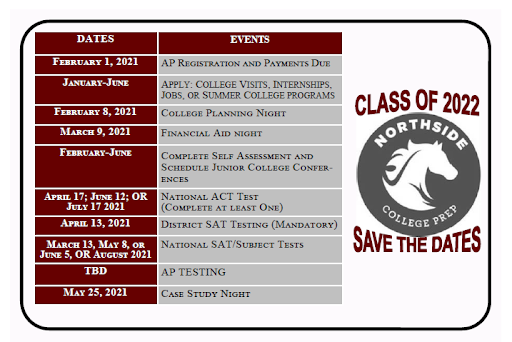table div table+table+table+table+table+table+table+table+table+table+table+table+table+table+table+table+table+table+table+table+table+table+table div table{width:100%;padding:0}table div table+table+table+table+table+table+table+table+table+table+table+table+table+table+table+table+table+table+table+table+table+table+table div table img{width:96.23%;padding:0;float:none}table div table+table+table+table+table+table+table+table+table+table+table+table+table+table+table+table+table+table+table+table+table+table+table div table td{width:100%;padding:0 1.88% 18px}/* styles */# UPDATE: Postsecondary Representative Visits

During the month of February, the counseling department scheduled virtual information sessions for summer enrichment programs, college access programs, as well as, colleges and universities. The virtual visits are available to students and parents to ensure all families have the opportunity to explore various postsecondary pathways and summer opportunities, engage with the representatives, and obtain answers to pertinent questions or concerns. Virtual visits are offered after school from 3:15 pm - 4:00 pm and during Flex Blocks 1-4 on Wednesdays.

To date, college, military, and GAP Year representatives are scheduled to connect with NCP students virtually, and the list is growing every day. Click HERE to view the list of upcoming and past postsecondary visits. Also, check out the messages from the admissions representatives and the additional resources they shared during their visits.

*Please note, all virtual visits will be conducted via CPS Google Meets and no email addresses outside CPS will be permitted into the virtual postsecondary visits. To protect the privacy and safety of students, parents will only be permitted to access virtual visits if their respective student is present and the student will be required to log in via their CPS Gmail account. All postsecondary visits will be managed and monitored by an NCP staff member.

 table div table+table+table+table+table+table+table+table+table+table+table+table+table+table+table+table+table+table+table+table+table+table+table+table+table div table{width:100%;padding:0}table div table+table+table+table+table+table+table+table+table+table+table+table+table+table+table+table+table+table+table+table+table+table+table+table+table div table img{width:96.23%;padding:0;float:none}table div table+table+table+table+table+table+table+table+table+table+table+table+table+table+table+table+table+table+table+table+table+table+table+table+table div table td{width:100%;padding:0 1.88% 18px}/* styles */# Counseling Department: ILP Naviance Curriculum

The CPS Office of School Counseling and Postsecondary Advising has created quarterly newsletters to continue to engage and support students and parents with their postsecondary planning, including: self-discovery, academic planning, and career and college exploration.

To view the newsletters for each grade level click on the links below. To access the counseling department college planning curriculum, students and parents can log into their Naviance account here to review and complete each task.

If you do not have access to your Naviance account, contact Ms. Sonia Fernandez at safernandez@cps.edu.

 table div table+table+table+table+table+table+table+table+table+table+table+table+table+table+table+table+table+table+table+table+table+table+table+table+table+table+table div table{width:100%;padding:0}table div table+table+table+table+table+table+table+table+table+table+table+table+table+table+table+table+table+table+table+table+table+table+table+table+table+table+table div table img{width:96.23%;padding:0;float:none}table div table+table+table+table+table+table+table+table+table+table+table+table+table+table+table+table+table+table+table+table+table+table+table+table+table+table+table div table td{width:100%;padding:0 1.88% 18px}/* styles */# CPS Scholarship Notifications and College Events Around the City

For more details about upcoming events and to access the most recent updates, click HERE to view the College Calendar on the Northside Prep Counseling website. Also, for additional scholarship opportunities, click HERE to visit the Northside College Prep Counseling page.

 table div table+table+table+table+table+table+table+table+table+table+table+table+table+table+table+table+table+table+table+table+table+table+table+table+table+table+table+table+table div table{width:100%;padding:0}table div table+table+table+table+table+table+table+table+table+table+table+table+table+table+table+table+table+table+table+table+table+table+table+table+table+table+table+table+table div table img{width:96.23%;padding:0;float:none}table div table+table+table+table+table+table+table+table+table+table+table+table+table+table+table+table+table+table+table+table+table+table+table+table+table+table+table+table+table div table td{width:100%;padding:0 1.88% 18px}/* styles */# SAT Updates from the College Board

As students and colleges adapt to new realities and changes in the college admissions process, the College Board is making sure our programs adapt with them.

The pandemic accelerated a process already underway at the College Board to simplify our work and reduce demands on students. As part of this process, we're making substantial investments in the SAT® Suite of Assessments and in tools to help colleges connect with students. We are providing three updates today and will continue to consult with our members on this ongoing work. Click here for more details about the updates.

 table div table+table+table+table+table+table+table+table+table+table+table+table+table+table+table+table+table+table+table+table+table+table+table+table+table+table+table+table+table+table+table div table{width:100%;padding:0}table div table+table+table+table+table+table+table+table+table+table+table+table+table+table+table+table+table+table+table+table+table+table+table+table+table+table+table+table+table+table+table div table img{width:96.23%;padding:0;float:none}table div table+table+table+table+table+table+table+table+table+table+table+table+table+table+table+table+table+table+table+table+table+table+table+table+table+table+table+table+table+table+table div table td{width:100%;padding:0 1.88% 18px}/* styles */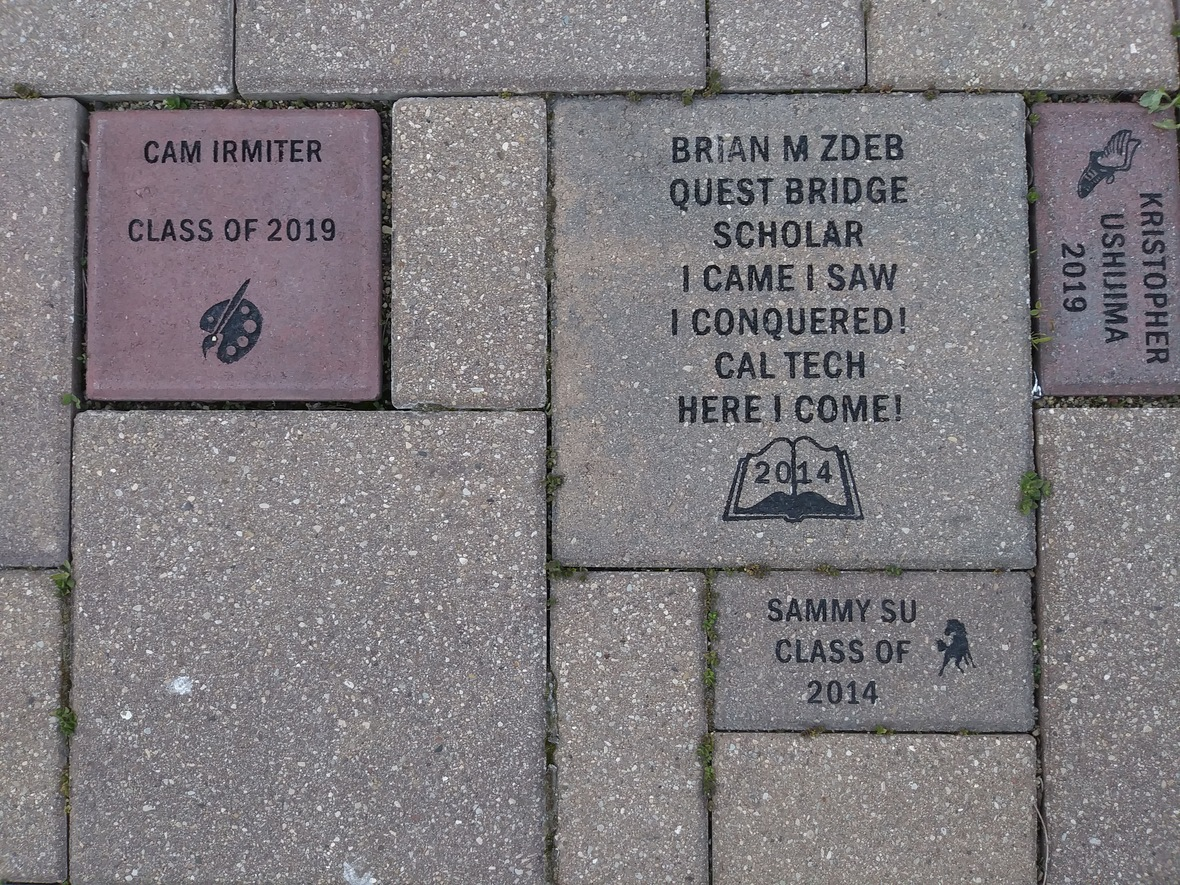# Custom NCP Brick With Your Personalized Message for Your Student!

Consider buying a custom NCP Brick for your child this year. Your brick, engraved with a personal message, will become a permanent part of the Northside College Prep Celestial Garden Walkway.

Your brick may be purchased in three sizes:

▪ 4" x 8" = \$50
▪ 8" x 8" = \$100
▪ 12" x 12" = \$150

Friends of Northside will provide a gift certificate acknowledging the purchase. Janet Jasmer will assist with the process and can answer any questions at jjasmer@atproperties.com.

Please click HERE and follow the payment and ordering instructions to have this lasting memory.

 table div table+table+table+table+table+table+table+table+table+table+table+table+table+table+table+table+table+table+table+table+table+table+table+table+table+table+table+table+table+table+table+table+table+table div table{width:100%;padding:0}table div table+table+table+table+table+table+table+table+table+table+table+table+table+table+table+table+table+table+table+table+table+table+table+table+table+table+table+table+table+table+table+table+table+table div table img{width:96.23%;padding:0;float:none}table div table+table+table+table+table+table+table+table+table+table+table+table+table+table+table+table+table+table+table+table+table+table+table+table+table+table+table+table+table+table+table+table+table+table div table td{width:100%;padding:0 1.88% 18px}/* styles */# Any Questions About NCP Communications Sent Via CNXT?

If you are having problems with SchoolCNXT, please contact the main office from 7:30 am - 3:15 pm at 773-534-3954, or you can email the NCP school clerk, Mary Grasz, at mtgrasz@cpu.edu.

NCP uses SchoolCNXT to communicate important information to parents and the school community. All families should have received a text message and/or email communication about signing up. If you did not receive that communication or need access to your student's account, you can gain access by using this tool on Northside's website.

 table div table+table+table+table+table+table+table+table+table+table+table+table+table+table+table+table+table+table+table+table+table+table+table+table+table+table+table+table+table+table+table+table+table+table+table+table+table div table{width:100%;padding:0}table div table+table+table+table+table+table+table+table+table+table+table+table+table+table+table+table+table+table+table+table+table+table+table+table+table+table+table+table+table+table+table+table+table+table+table+table+table div table img{width:96.23%;padding:0;float:none}table div table+table+table+table+table+table+table+table+table+table+table+table+table+table+table+table+table+table+table+table+table+table+table+table+table+table+table+table+table+table+table+table+table+table+table+table+table div table td{width:100%;padding:0 1.88% 18px}/* styles */# Winter Sports Update

CPS has approved the winter sports season to resume practices for the following sports: boys/girls basketball, boys/girls bowling, cheerleading, competitive dance, and boys swimming & diving. Badminton is also beginning as it has been moved to the winter season.

In the next few days, we will be announcing tryouts and practice dates for our winter sports.

## Winter Sports Opportunities

The following winter sports are still accepting registrations and will have tryouts: Badminton, Boys/Girls Bowling, and Boys/Girls Basketball. Students interested in participating in these sports must register so that coaches can communicate the tryout schedule. See below for information on how to register. Tryouts for boys swimming, competitive dance, and competitive cheer were conducted before winter sports were paused back in November.

## Sports Registration

Visit the NCP athletics website HERE and click on the Activity Registration tab. In addition to registering, students must have a current physical on file before they try out or practice. Contact athletic director Henry Henderson at hhenderson@cps.edu for more information.

The athletic department will publish tryout and practice information in the next few days as it becomes available. Be sure to look for a message via SchoolCNXT and check the NCP athletic website for any updates.

 table div table+table+table+table+table+table+table+table+table+table+table+table+table+table+table+table+table+table+table+table+table+table+table+table+table+table+table+table+table+table+table+table+table+table+table+table+table+table+table div table{width:100%;padding:0}table div table+table+table+table+table+table+table+table+table+table+table+table+table+table+table+table+table+table+table+table+table+table+table+table+table+table+table+table+table+table+table+table+table+table+table+table+table+table+table div table img{width:96.23%;padding:0;float:none}table div table+table+table+table+table+table+table+table+table+table+table+table+table+table+table+table+table+table+table+table+table+table+table+table+table+table+table+table+table+table+table+table+table+table+table+table+table+table+table div table td{width:100%;padding:0 1.88% 18px}/* styles */# Photo Submissions Requested for NCP Stampede!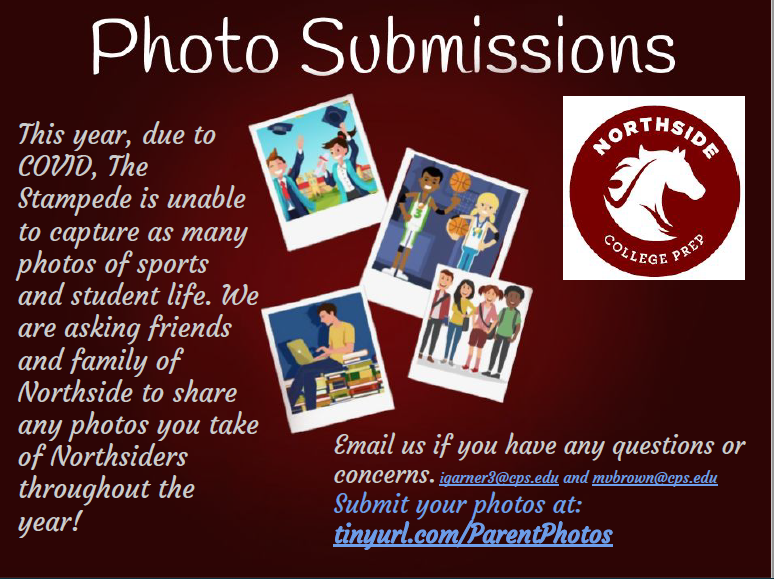# 2020-2021 Yearbook Ads for Seniors Now Available

Seniors now have the opportunity to place ads in the 2020-2021 yearbook by visiting the yearbook website. After clicking on "continue", click on "start your ad" to begin.

 table div table+table+table+table+table+table+table+table+table+table+table+table+table+table+table+table+table+table+table+table+table+table+table+table+table+table+table+table+table+table+table+table+table+table+table+table+table+table+table+table+table+table+table div table td,table.module-42{width:100%;padding:0}table div table+table+table+table+table+table+table+table+table+table+table+table+table+table+table+table+table+table+table+table+table+table+table+table+table+table+table+table+table+table+table+table+table+table+table+table+table+table+table+table+table+table+table div table{width:100%;float:none;margin-left:auto;margin-right:auto;padding:0}table div table+table+table+table+table+table+table+table+table+table+table+table+table+table+table+table+table+table+table+table+table+table+table+table+table+table+table+table+table+table+table+table+table+table+table+table+table+table+table+table+table+table+table div table a{border:0 none;text-decoration:none}table div table+table+table+table+table+table+table+table+table+table+table+table+table+table+table+table+table+table+table+table+table+table+table+table+table+table+table+table+table+table+table+table+table+table+table+table+table+table+table+table+table+table+table div table img{width:100%!important;border:0 none;text-decoration:none}/* styles */

# Update on school portraits for 9th, 10th, and 11th graders

This letter was sent in CNXT explaining the process for submitting your student's school portrait to be included in the yearbook. The NCP code is 21740 if needed. If you have any questions, contact Ms. McCreedy at mkmccreedy@cps.edu.

# Order a Yearbook Today!

Interested in purchasing a copy of the 2020-2021 Stampede, NCP's yearbook? Click HERE to start your order! And if you didn't get a chance to purchase a copy of the 2019-2020 yearbook, you now have a change to buy one of those, too! Just email Ms. McCreedy at mkmccreedy@cps.edu after you've completed your 2020-2021 order.

Copies are \$45 until March 1 and \$55 afterwards.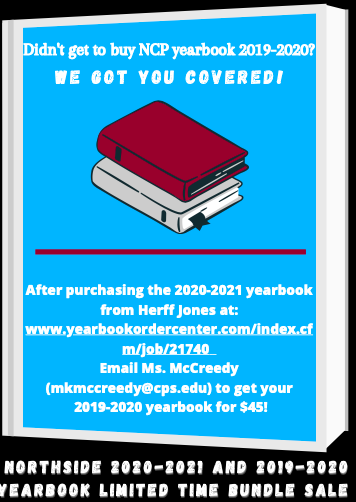table div table+table+table+table+table+table+table+table+table+table+table+table+table+table+table+table+table+table+table+table+table+table+table+table+table+table+table+table+table+table+table+table+table+table+table+table+table+table+table+table+table+table+table+table+table+table div table{width:100%;padding:0}table div table+table+table+table+table+table+table+table+table+table+table+table+table+table+table+table+table+table+table+table+table+table+table+table+table+table+table+table+table+table+table+table+table+table+table+table+table+table+table+table+table+table+table+table+table+table div table img{width:96.23%;padding:0;float:none}table div table+table+table+table+table+table+table+table+table+table+table+table+table+table+table+table+table+table+table+table+table+table+table+table+table+table+table+table+table+table+table+table+table+table+table+table+table+table+table+table+table+table+table+table+table+table div table td{width:100%;padding:0 1.88% 18px}/* styles */# Do you have news or team results to share with the Northside parent community?

 table div table+table+table+table+table+table+table+table+table+table+table+table+table+table+table+table+table+table+table+table+table+table+table+table+table+table+table+table+table+table+table+table+table+table+table+table+table+table+table+table+table+table+table+table+table+table+table+table+table div table{width:100%;padding:0}table div table+table+table+table+table+table+table+table+table+table+table+table+table+table+table+table+table+table+table+table+table+table+table+table+table+table+table+table+table+table+table+table+table+table+table+table+table+table+table+table+table+table+table+table+table+table+table+table+table div table img{width:96.23%;padding:0;float:none}table div table+table+table+table+table+table+table+table+table+table+table+table+table+table+table+table+table+table+table+table+table+table+table+table+table+table+table+table+table+table+table+table+table+table+table+table+table+table+table+table+table+table+table+table+table+table+table+table+table div table td{width:100%;padding:0 1.88% 18px}/* styles */# Support Northside - Shop AmazonSmile

AmazonSmile is a simple and automatic way to support Northside every time you shop.

Please note that our AmazonSmile name is Friends of "North" "Side" (North Side - two words at AmazonSmile).

 table div table+table+table+table+table+table+table+table+table+table+table+table+table+table+table+table+table+table+table+table+table+table+table+table+table+table+table+table+table+table+table+table+table+table+table+table+table+table+table+table+table+table+table+table+table+table+table+table+table+table+table div table{width:100%;padding:0}table div table+table+table+table+table+table+table+table+table+table+table+table+table+table+table+table+table+table+table+table+table+table+table+table+table+table+table+table+table+table+table+table+table+table+table+table+table+table+table+table+table+table+table+table+table+table+table+table+table+table+table div table img{width:96.23%;padding:0;float:none}table div table+table+table+table+table+table+table+table+table+table+table+table+table+table+table+table+table+table+table+table+table+table+table+table+table+table+table+table+table+table+table+table+table+table+table+table+table+table+table+table+table+table+table+table+table+table+table+table+table+table+table div table td{width:100%;padding:0 1.88% 18px}/* styles */# Follow Us on Social Media

▪ NCP's Official Facebook Page
▪ Northside Prep Parents Facebook Page, a closed group for parents, by parents, to share their knowledge of Northside
▪ NCP's Twitter Page
▪ Friends of Northside Facebook Page, for news everyone can view
 ▪ Northside Prep Parents Facebook Page, a closed group for parents, by parents, to share their knowledge of Northside
 ▪ Friends of Northside Facebook Page, for news everyone can view
 table div table+table+table+table+table+table+table+table+table+table+table+table+table+table+table+table+table+table+table+table+table+table+table+table+table+table+table+table+table+table+table+table+table+table+table+table+table+table+table+table+table+table+table+table+table+table+table+table+table+table+table+table+table div table{width:100%;padding:0}table div table+table+table+table+table+table+table+table+table+table+table+table+table+table+table+table+table+table+table+table+table+table+table+table+table+table+table+table+table+table+table+table+table+table+table+table+table+table+table+table+table+table+table+table+table+table+table+table+table+table+table+table+table div table img{width:96.23%;padding:0;float:none}table div table+table+table+table+table+table+table+table+table+table+table+table+table+table+table+table+table+table+table+table+table+table+table+table+table+table+table+table+table+table+table+table+table+table+table+table+table+table+table+table+table+table+table+table+table+table+table+table+table+table+table+table+table div table td{width:100%;padding:0 1.88% 18px}/* styles */table div table+table+table+table+table+table+table+table+table+table+table+table+table+table+table+table+table+table+table+table+table+table+table+table+table+table+table+table+table+table+table+table+table+table+table+table+table+table+table+table+table+table+table+table+table+table+table+table+table+table+table+table+table+table div table,table.module-53{width:49.25%;float:left;padding:0}table div table+table+table+table+table+table+table+table+table+table+table+table+table+table+table+table+table+table+table+table+table+table+table+table+table+table+table+table+table+table+table+table+table+table+table+table+table+table+table+table+table+table+table+table+table+table+table+table+table+table+table+table+table+table div table a{border:0 none;text-decoration:none}table div table+table+table+table+table+table+table+table+table+table+table+table+table+table+table+table+table+table+table+table+table+table+table+table+table+table+table+table+table+table+table+table+table+table+table+table+table+table+table+table+table+table+table+table+table+table+table+table+table+table+table+table+table+table div table img{width:100%!important;border:0 none;text-decoration:none}table div table+table+table+table+table+table+table+table+table+table+table+table+table+table+table+table+table+table+table+table+table+table+table+table+table+table+table+table+table+table+table+table+table+table+table+table+table+table+table+table+table+table+table+table+table+table+table+table+table+table+table+table+table+table div table td{width:100%;padding:0 20px 20px 0}/* styles */ To view this message in your browser, click HERE. Freshmen, click HERE for the Class of 2024 web page. Northside’s website can be found HERE. Friends of Northside's website can be found HERE. To see all volunteer opportunities on one page, click HERE. To ensure delivery of This Week at Northside, please add Thisweek@friendsofnorthside.org to your address book. Please do not reply to this e-mail. Send your questions or comments to Friends of Northside.
 table div table+table+table+table+table+table+table+table+table+table+table+table+table+table+table+table+table+table+table+table+table+table+table+table+table+table+table+table+table+table+table+table+table+table+table+table+table+table+table+table+table+table+table+table+table+table+table+table+table+table+table+table+table+table+table div table{width:100%;padding:0}table div table+table+table+table+table+table+table+table+table+table+table+table+table+table+table+table+table+table+table+table+table+table+table+table+table+table+table+table+table+table+table+table+table+table+table+table+table+table+table+table+table+table+table+table+table+table+table+table+table+table+table+table+table+table+table div table img{width:96.23%;padding:0;float:none}table div table+table+table+table+table+table+table+table+table+table+table+table+table+table+table+table+table+table+table+table+table+table+table+table+table+table+table+table+table+table+table+table+table+table+table+table+table+table+table+table+table+table+table+table+table+table+table+table+table+table+table+table+table+table+table div table td{width:100%;padding:0 1.88% 18px}/* styles *//* styles */

## SIGN ME UP for This Week at Northside

 table div table+table+table+table+table+table+table+table+table+table+table+table+table+table+table+table+table+table+table+table+table+table+table+table+table+table+table+table+table+table+table+table+table+table+table+table+table+table+table+table+table+table+table+table+table+table+table+table+table+table+table+table+table+table+table+table+table+table div table{width:100%;padding:0}table div table+table+table+table+table+table+table+table+table+table+table+table+table+table+table+table+table+table+table+table+table+table+table+table+table+table+table+table+table+table+table+table+table+table+table+table+table+table+table+table+table+table+table+table+table+table+table+table+table+table+table+table+table+table+table+table+table+table div table img{width:96.23%;padding:0;float:none}table div table+table+table+table+table+table+table+table+table+table+table+table+table+table+table+table+table+table+table+table+table+table+table+table+table+table+table+table+table+table+table+table+table+table+table+table+table+table+table+table+table+table+table+table+table+table+table+table+table+table+table+table+table+table+table+table+table+table div table td{width:100%;padding:0 1.88% 18px}/* styles */